# Solving Problems with Systems of Equations Chapter Exam

Exam Instructions:

Choose your answers to the questions and click 'Next' to see the next set of questions. You can skip questions if you would like and come back to them later with the yellow "Go To First Skipped Question" button. When you have completed the practice exam, a green submit button will appear. Click it to see your results. Good luck!

### Page 3

#### Question 12 12. Which of the following is a solution to the system of linear equations shown?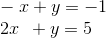#### Question 14 14. A graph of a system of linear equations is shown along with the system. What is the solution to the system?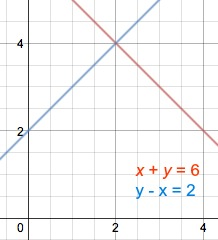### Page 5

#### Question 23 23. The graphs of f(x) = 4x - 5 and g(x) = -x are shown along with their intersection point (1,-1). Based on this, what value of x will make f(x) = g(x)?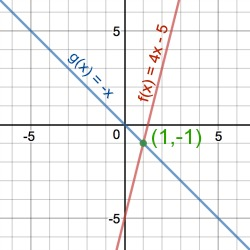### Page 6

#### Question 27 27. Look at the graph. What is the solution to the system of equations?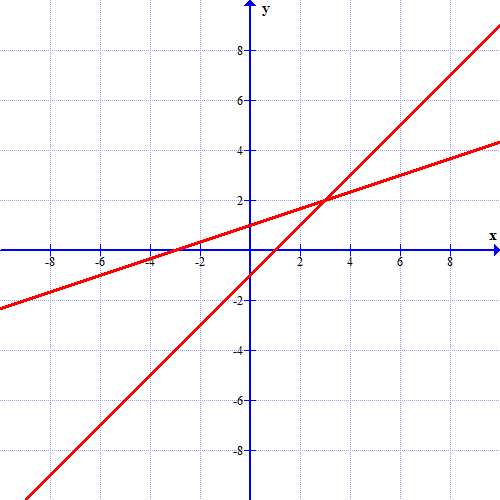#### Question 29 29. What is the solution to the system of equations shown in the graph below?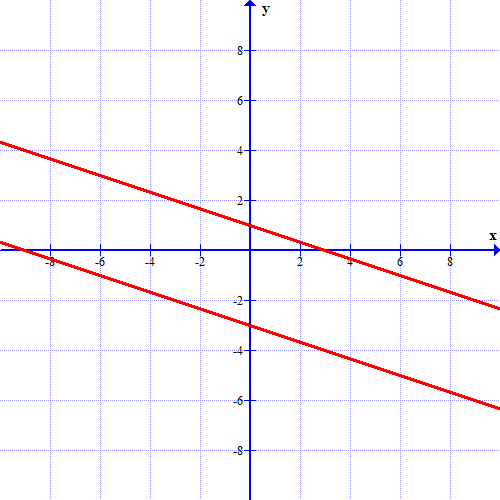#### Solving Problems with Systems of Equations Chapter Exam Instructions

Choose your answers to the questions and click 'Next' to see the next set of questions. You can skip questions if you would like and come back to them later with the yellow "Go To First Skipped Question" button. When you have completed the practice exam, a green submit button will appear. Click it to see your results. Good luck!

Support